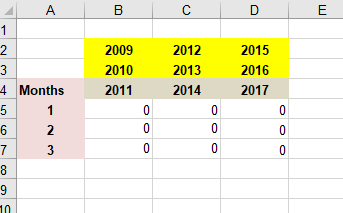VBA modification from earlier solution by Rgonzo1971

I had this question after viewing Simple Macro but is a big challenge. any Expert can solve this please?.

Rgonzo1971 helped me alot. thanks to him.

he helped me with the code below.

``````Sub MacroAll()
Dim Main As Worksheet

Set Main = Sheets("Main")
Set ShD = Sheets("NewData")

intYearCol = Range("dataYear").Column
intMonthCol = Range("dataMonth").Column
intProductCol = Range("dataPRODUCT").Column
intAmountCol = Range("dataAMOUNT").Column

For Each cl In Range(Main.Range("B3"), Main.Range("D3"))
mySum = 0
Application.StatusBar = "Processing month " & Main.Cells(cl.Row, 1).Value & " for year " & Main.Cells(2, cl.Column).Value
For Each c In Range(ShD.Range("a2"), ShD.Range("a" & Rows.Count).End(xlUp))

If ShD.Cells(c.Row, intYearCol).Value = (Main.Cells(2, cl.Column).Value2 * 1) And ShD.Cells(c.Row, intMonthCol).Value = (Main.Range("A" & cl.Row).Value2 * 1) _
And ShD.Cells(c.Row, intMonthCol).Value <> 111 And _
ShD.Cells(c.Row, intProductCol).Value Like "[5-7]*" Then
mySum = mySum + ShD.Cells(c.Row, intAmountCol).Value
End If
Next
cl.Value = mySum
Next

Application.StatusBar = False

End Sub
``````

I wanted to modify the code, so that it takes the years from the upper 3 rows on the columns B C D

i modified the code like this, but it does not work and i do not know how to include the other 2 upper columns highlighted in yellow into the codeAttached is the workbook.

``````Sub MacroAll()
Dim Main As Worksheet

Set Main = Sheets("Main")
Set ShD = Sheets("NewData")

intYearCol = Range("dataYear").Column
intMonthCol = Range("dataMonth").Column
intProductCol = Range("dataPRODUCT").Column
intAmountCol = Range("dataAMOUNT").Column

For Each cl In Range(Main.Range("B3"), Main.Range("D3"))
mySum = 0
Application.StatusBar = "Processing month " & Main.Cells(cl.Row, 1).Value & " for year " & Main.Cells(2, cl.Column).Value
For Each c In Range(ShD.Range("a2"), ShD.Range("a" & Rows.Count).End(xlUp))

If ShD.Cells(c.Row, intYearCol).Value = (Main.Cells(2, cl.Column).Value2 * 1) And ShD.Cells(c.Row, intMonthCol).Value = (Main.Range("A" & cl.Row).Value2 * 1) _
And ShD.Cells(c.Row, intMonthCol).Value <> 111 And _
ShD.Cells(c.Row, intProductCol).Value Like "[5-7]*" Then
mySum = mySum + ShD.Cells(c.Row, intAmountCol).Value
End If
Next
cl.Value = mySum
Next

Application.StatusBar = False

End Sub
``````
WE.xlsb
Who is Participating?
I wear a lot of hats...

"The solutions and answers provided on Experts Exchange have been extremely helpful to me over the last few years. I wear a lot of hats - Developer, Database Administrator, Help Desk, etc., so I know a lot of things but not a lot about one thing. Experts Exchange gives me answers from people who do know a lot about one thing, in a easy to use platform." -Todd S.

CEOCommented:
Try this:

``````Sub MacroAll()
Dim Main As Worksheet

Set Main = Sheets("Main")
Set ShD = Sheets("NewData")

intYearCol = Range("dataYear").Column
intMonthCol = Range("dataMonth").Column
intProductCol = Range("dataPRODUCT").Column
intAmountCol = Range("dataAMOUNT").Column

For Each cl In Range(Main.Range("B5"), Main.Range("D7"))
mySum = 0
Application.StatusBar = "Processing month " & Main.Cells(cl.Row, 1).Value & " for year " & Main.Cells(2, cl.Column).Value
For Each c In Range(ShD.Range("a2"), ShD.Range("a" & Rows.Count).End(xlUp))

If (ShD.Cells(c.Row, intYearCol).Value = (Main.Cells(2, cl.Column).Value2 * 1) _
Or ShD.Cells(c.Row, intYearCol).Value = (Main.Cells(3, cl.Column).Value2 * 1) _
Or ShD.Cells(c.Row, intYearCol).Value = (Main.Cells(4, cl.Column).Value2 * 1)) _
And ShD.Cells(c.Row, intMonthCol).Value = (Main.Range("A" & cl.Row).Value2 * 1) _
And ShD.Cells(c.Row, intMonthCol).Value <> 111 And _
ShD.Cells(c.Row, intProductCol).Value Like "[5-7]*" Then
mySum = mySum + ShD.Cells(c.Row, intAmountCol).Value
End If
Next
cl.Value = mySum
Next

Application.StatusBar = False

End Sub
``````

Experts Exchange Solution brought to you by